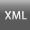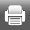Volume 9, Issue 1 (Winter 2014)J. Mon. Ec. 2014, 9(1): 1-30 Back to browse issues page
Optimal Portfolio Selection for Tehran Stock Exchange Using Conditional, Partitioned and Worst-case Value at Risk Measures
1- university of Tehran
Abstract:   (2229 Views)

This paper presents an optimal portfolio selection approach based on value at risk (VaR), conditional value at risk (CVaR), worst-case value at risk (WVaR) and partitioned value at risk (PVaR) measures as well as calculating these risk measures. Mathematical solution methods for solving these optimization problems are inadequate and very complex for a portfolio with high number of assets. For these reasons, a combination of particle swarm optimization (PSO) and genetic algorithm (GA) is used to determine optimized weights of assets. Stocks’ Optimized weight results show that proposed algorithm gives more accurate outcomes in comparison with GA algorithm. According to back-testing analysis, PVaR and WVaR overestimate risk value while VaR and CVaR give a rather accurate estimation. A set of companies in Tehran Stock Exchange are considered as a case study for empirical analysis.

JEL Classification: G10, G11, G19

Keywords: portfolio optimization, value at risk, CVaR, WVaR, PVaR, HGAPSO